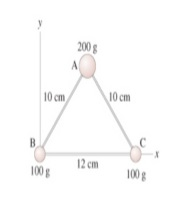Get help with parametric equations

Recent questions in Parametric equationsmaduregimc 2021-12-22 Answered

Find the maximum rate of change of f at the given point and the direction in which it occurs. $$\displaystyle{f{{\left({x},{y},{z}\right)}}}=\sqrt{{{x}^{{2}}+{y}^{{2}}+{z}^{{2}}}},\ {\left({9},{7},-{2}\right)}$$ maximum rate of change = direction vector =Wanda Kane 2021-12-20 Answered

Find two unit vectors orthogonal to both $$\displaystyle{j}-{k}$$ and $$\displaystyle{i}+{j}$$.Julia White 2021-12-20 Answered

If a curve has the property that the position vector $$\displaystyle{r}{\left({t}\right)}$$ is always perpendicular to the tangent vector $$\displaystyle{r}'{\left({t}\right)}$$, show that the curve lies on a sphere with center the origin.namenerk 2021-12-18 Answered

What is the difference between f(-x) and -f(x)? What is the difference between $$\displaystyle{f{{\left(-{x}\right)}}}\ {\quad\text{and}\quad}\ -{f{{\left({x}\right)}}}$$ in terms of their graphs?Sandra Allison 2021-12-16 Answered

How do you find parametric equations for the tangent line to the curve with the given parametric equations $$\displaystyle{x}={7}{t}^{{2}}-{4}$$ and $$\displaystyle{y}={7}{t}^{{2}}+{4}$$ and $$\displaystyle{z}={6}{t}+{5}$$ and $$\displaystyle{\left({3},{11},{11}\right)}$$?Mabel Breault 2021-12-15 Answered

Find parametric equations for the path a particle that moves along the circle $$\displaystyle{x}^{{{2}}}+{\left({y}-{1}\right)}^{{{2}}}={4}$$ Find parametric equations for the path a particle that moves along the circle $$\displaystyle{x}^{{{2}}}+{\left({y}-{1}\right)}^{{{2}}}={4}$$. In the manner describe a) One around clockwise starting at (2,1) b) Three times around counterclockwise starting at (2,1) c) halfway around counterclockwise starting at (0,3)Charles Kingsley 2021-12-15 Answered

The three masses shown in the figure are connected by massless, rigid rods. Find the moment of inertia about an axis that passes through mass A and is perpendicular to the page. Express your answer to two significant figures and include the appropriate units. Find the moment of inertia about an axis that passes through masses B and C. Express your answer to two significant figures and include the appropriate units.chezmarylou1i 2021-12-14 Answered

I am struggling with the concept of parameterizing curves. I am not even sure if I know what it means so I tried to look some things up. Since I didn't know what a parametric equation was I also looked that up: In mathematics, parametric equations of a curve express the coordinates of the points of the curve as functions of a variable, called a parameter. For example, $$\displaystyle{x}={\cos{}}$$ $$\displaystyle{y}={\sin{}}$$ So, basically I have some curve and I want to make up some equation that describes that curve? But doesn't this get very difficult if you don't have simple circles or spirals? Maybe someone can explain to me what's going on here. Tha in advance.aspifsGak5u 2021-12-14 Answered

Find the maximum and minimum values attained by the function f along the path $$\displaystyle{c}{\left({t}\right)}$$. $$\displaystyle{\left({a}\right)}{f{{\left({x},{y}\right)}}}={x}{y};{c}{\left({t}\right)}={\left({\cos{{\left({t}\right)}}},{\sin{{\left({t}\right)}}}\right)};{0}\le{t}\le{2}\pi$$ maximum value__________ minimum value__________ (b) $$\displaystyle{f{{\left({x},{y}\right)}}}={x}^{{{2}}}+{y}^{{{2}}};{c}{\left({t}\right)}={\left({\cos{{\left({t}\right)}}},{8}{\sin{{\left({t}\right)}}}\right)};{0}\le{t}\le{2}\pi$$ maximum value__________ minimum value__________Margie Marx 2021-12-14 Answered

What is the meaning of 'Sxx' and 'Sxy' in simple linear regression? I know the formula but what is the meaning of those formulas?lugreget9 2021-12-14 Answered

Vector $$\displaystyle\vec{{{A}}}$$ is 3.00 units in length and points along the positive x-axis. Vector $$\displaystyle\vec{{{B}}}$$ is 4.00 units in tength and points along the negative y-axis. Use graphical methods to find the magnitude and direction of the vectors $$\displaystyle\vec{{{A}}}+\vec{{{B}}}$$sputneyoh 2021-12-13 Answered

Find the maximum rate of change of f at the given point and the direction in which it occurs. $$\displaystyle{f{{\left({x},{y}\right)}}}={4}{y}\sqrt{{{x}}},\ {\left({4},{9}\right)}$$ maximum rate of change = direction vector =David Lewis 2021-12-11 Answered

Find the equation of the tan line to the curve at $$\displaystyle{\left({81};{9}\right)}$$ of $$\displaystyle{y}=\sqrt{{{x}}}$$sputneyoh 2021-12-10 Answered

What is the equation of the line to the parabola $$\displaystyle{y}={x}^{{{2}}}+{5}{x}+{1}$$ through the point at $$\displaystyle{\left({\frac{{{1}}}{{{2}}}},\ {\frac{{{15}}}{{{4}}}}\right)}$$?expeditiupc 2021-12-10 Answered

Evaluate the vector-valued function at each given value of t. $$\displaystyle{r}{\left({t}\right)}={\left({2}{t}+{1}\right)}{i}+{t}^{{{2}}}{j}-\sqrt{{t}}+{2}{k}$$ (a) $$\displaystyle{r}{\left({0}\right)}{\left({b}\right)}{r}{\left\lbrace-{2}\right)}{\left({c}\right)}{r}{\left({c}-{1}\right)}$$ (d) $$\displaystyle{r}{\left({1}+\triangle{t}\right)}-{r}{\left({1}\right)}$$tebollahb 2021-12-10 Answered

Find the domain of the vector-valued functions: (a) $$\displaystyle\vec{{{r}}}{\left({t}\right)}={\left\langle{t}^{{-{1}}},{\left({t}+{1}\right)}^{{-{1}}},{{\sin}^{{-{1}}}{\left({t}\right)}}\right\rangle}$$ (b) $$\displaystyle\vec{{{r}}}{\left({t}\right)}={\left\langle\sqrt{{{8}-{t}^{{{3}}}}},{I}{n}{\left({t}\right)},{e}^{{\sqrt{{{t}}}}}\right\rangle}$$Kelly Nelson 2021-12-08 Answered

Find the domain of the vector-valued function $$\displaystyle{r}{\left({t}\right)}={F}{\left({t}\right)}\times{G}{\left({t}\right)}$$, where $$\displaystyle{F}{\left({t}\right)}={\sin{{t}}}{i}+{\cos{{t}}}{j},{G}{\left({t}\right)}={\sin{{t}}}{j}+{\cos{{t}}}{k}$$Russell Gillen 2021-12-07 Answered

Suppose parametric equations for the line segment between $$\displaystyle{6},\ {7}{)}$$ and $$\displaystyle{\left({4},\ {5}\right)}$$ have the form: $\begin{cases}x(t) =a= bt \\ y(t)=c+dt \end{cases}$ If the parametric curve starts at $$\displaystyle{\left({6},\ {7}\right)}$$ when $$\displaystyle{t}={0}$$ and ends at $$\displaystyle{\left({4},\ {5}\right)}$$ at $$\displaystyle{t}={1}$$, then find $$\displaystyle{a},\ {b},\ {c}$$ and d.Anne Wacker 2021-12-07 Answered

Equation of a line and a line segment Let $$\displaystyle{e}{l}{l}$$ be the line that passes through the points $$\displaystyle{P}_{{{0}}}{\left(-{3},\ {5},\ {8}\right)}$$ and $$\displaystyle{P}_{{{1}}}{\left({4},\ {2},\ -{1}\right)}$$. a. Find an equation of $$\displaystyle{e}{l}{l}$$. b. Find parametric equations of the line segment that extends from $$\displaystyle{P}_{{{0}}}$$ to $$\displaystyle{P}_{{{1}}}$$.eozoischgc 2021-12-07 Answered

...